### Some experiments with Local Linear Regression. Part I – A primer on Local Linear Regression

Local Linear Pt. 1

## Introduction¶

In today's Machine Learning world, Linear Regression often appears to fall short of other more advanced techniques due to its simpilictiy and hence supposed low accuracy for complex problems. On the other hand, linear type algorithms can have a bunch of advantages that make them interesting choices nonetheless - interpretability of the model, convexity of the loss function or less proneness to overfitting to name a few. I have recently experimented a little with Local Linear Regression as a quite powerful option if done with some thought.

## What is Local Linear Regression?¶

Standard Linear Regression algorithms typically fit a simple hyperplane through our data trying to minimize some error criterion. The obvious drawback comes when our data is rather non-linear and Linear Regression is unable to account for anything beyond linearity.. At this point, it might already be tempting to pull out your typical ML toolbox and use an advanced non-linear technique like Boosting, Random Forests or even Deep Learning. Statisticians of the early days were already facing this problem way before the days of modern Machine Learning and came up with - in my opinion - quite smart solutions. One of those techniques is said Local Regression and a look at the Wiki references tells us that it is indeed around 40 years old.

The idea of Local Linear Regression approaches is to not try fitting a hyperplane through the whole dataset but only through a small neighborhood around a point we are interested in. Depending on how smooth the function we are trying to approximate is we might end up with quite good results. Let's look at an example to exemplify the matter further:

Consider a mere cubic relationship:

$$y=0.5\cdot x^3$$

For the remainder of this post, I will only consider regression problems. Nevertheless, the approach can easily be applied to classification as well by replacing the Linear Regression model by a linear classification model.

In :
import numpy as np
import matplotlib.pyplot as plt

In :
x = np.linspace(-3,3, 100)
y = 0.5*x**3

plt.figure(figsize = (8,8))
plt.plot(x,y, lw = 3)
plt.xlim((-3,3))
plt.ylim((np.min(y), np.max(y)))
plt.grid()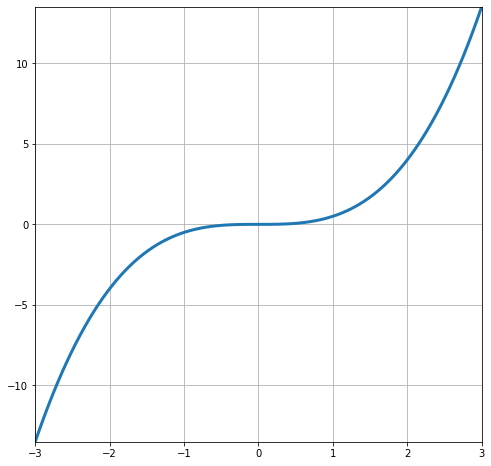According to Taylor's Theorem we can approximate an appropriately differentiable function via

$$f(x)\approx f(a)+f'(a)(x-a)$$

This approximation is obviously linear and we can show the results for our example:

In :
def approx_function(a):

return lambda x: 0.5*a**3 + 1.5*a**2*(x-a)

a1 = 0.
a2 = -2.
a3 = 1.5

f1 = approx_function(a1)(x)
f2 = approx_function(a2)(x)
f3 = approx_function(a3)(x)

plt.figure(figsize = (8,8))
plt.plot(x,y, lw = 3)
plt.plot(x,f1, linestyle = '--', color = "orange", lw = 2)
plt.plot(x,f2, linestyle = '--', color = "green", lw = 2)
plt.plot(x,f3, linestyle = '--', color = "red", lw = 2)

plt.scatter(a1, 0.5*a1**3, color = "orange", s = 75)
plt.scatter(a2, 0.5*a2**3, color = "green", s = 75)
plt.scatter(a3, 0.5*a3**3, color = "red", s = 75)

plt.annotate(str(a1)+","+str(0.5*a1**3), (a1+0.05, 0.5*a1**3+0.2), fontsize=15)
plt.annotate(str(a2)+","+str(0.5*a2**3), (a2+0.05, 0.5*a2**3+0.2), fontsize=15)
plt.annotate(str(a3)+","+str(np.round(0.5*a3**3,1)), (a3+0.05, 0.5*a3**3+0.2), fontsize=15)

plt.xlim((-3,3))
plt.ylim((np.min(y), np.max(y)))
plt.grid()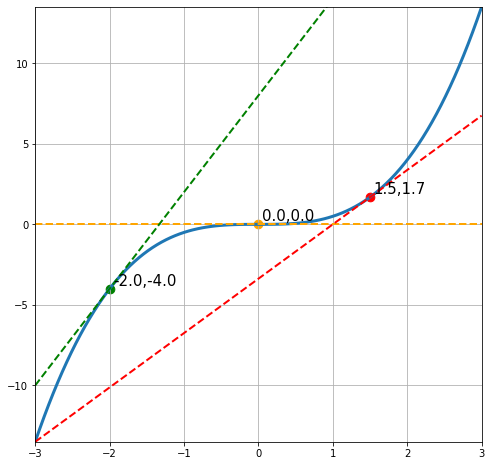If you look at the lines, you see that the line at $a=0$ is a quite reasonable approximation for $f(x)$ inside the interval $x\in[-0.5,0.5]$. The other lines on the other hand lose their predictive power much faster the further we move away from $a$. However, the approximation at $a$ itself is always perfect by definition.

This approach can be used analogously in a regression and classification context except that now we need to estimate the optimal linear line in the neighborhood of our target point. To get some intuition, suppose we had some data points that were sampled along our function and our goal is to estimate the function value at $x=-2.0$:

In :
np.random.seed(321)
x_sample = np.random.uniform(-3,3,size=(50,))
y_sample = 0.5*x_sample**3

plt.figure(figsize = (8,8))
plt.plot(x,y, lw = 3, zorder = 0)
plt.scatter(x_sample, y_sample, color = "orange", s = 50, zorder = 5)
plt.scatter(-2,-4, color = "red", s=200, zorder = 10)

plt.annotate(str(-2.0)+","+str("?.?"), (-2+0.05, 0.5*-2**3+0.2), fontsize=20, zorder = 20)

plt.xlim((-3,3))
plt.ylim((np.min(y), np.max(y)))
plt.grid()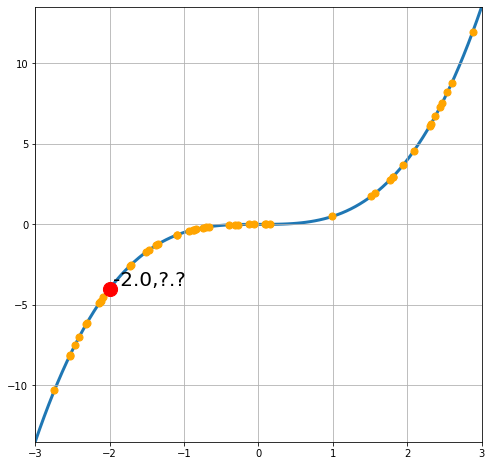The first question here is: How can our Linear Regression model approximate the target function in the neighborhood of our target datapoint? For this to happen we want to penalize model errors stronger for those points that are closer to the target point $x_{target}$. Technically, we have a per-observation loss

$$\tilde{L}(f(x_i),y_i)) = L(f(x_i), y_i)\cdot s(x_i,x_{target})$$

where $s(\cdot, \cdot)$ is some measure of proximity between two points. For example, we could choose

$$s(x_i,x_{target})=e^{-||x_i-x_{target}||_2}$$

i.e we use the exponential of the negative Euclidean Distance between the two points. Let's do this calculation for our example:

In :
from sklearn.metrics import pairwise_distances

target = np.array(-2).reshape(1,1)
similarities = np.exp(-pairwise_distances(x_sample.reshape(-1,1), target))

In :
plt.figure(figsize = (8,8))
plt.scatter(x_sample, similarities.reshape(-1))
plt.vlines(-2, ymin = 0, ymax = 1, color = "red", linestyle = "--")

plt.xlim((-3,3))
plt.ylim((0,1))

plt.grid()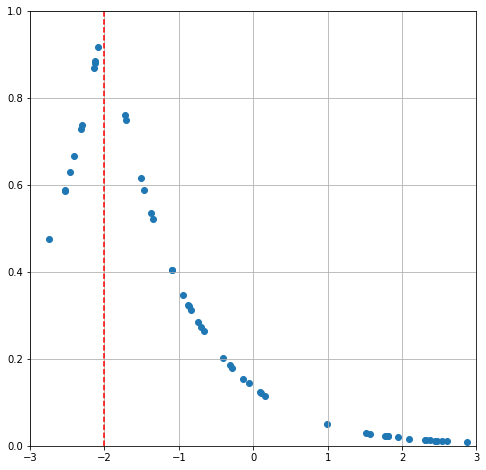As we would expect, the proximities are highest for points around the target $x=-2.0$ and decline as we move away from it in either direction.

Next step now is to add the similarity information as weights into a Linear Regression model - i.e. we use the typical Mean Squared Error (MSE) as the base loss. Thankfully, scikit-learn provides the necessary interface for a weighted MSE loss:

In :
from sklearn.linear_model import LinearRegression

In :
model = LinearRegression()
model.fit(x_sample.reshape(-1,1), y_sample.reshape(-1,1), sample_weight = similarities.reshape(-1))

print("Predicted value at x={}: {}".format(target[0,0],model.predict(target)[0,0]))

Predicted value at x=-2: -4.706809010226895

In :
np.random.seed(123)

x = np.linspace(-3,3, 100)
y = 0.5*x**3

plt.figure(figsize = (8,8))
plt.plot(x,y, label = "Target function")
plt.plot(x,f2, linestyle = '--', color = "green", lw = 2, label = "Tangent line")
plt.plot(x,model.predict(x.reshape(-1,1)), linestyle=':', color = "purple", lw=2, label = "Predicted tangent")
plt.scatter(a2, 0.5*a2**3, color = "green", s = 75)

plt.xlim((-3,3))
plt.ylim((np.min(y), np.max(y)))
plt.legend()

plt.grid()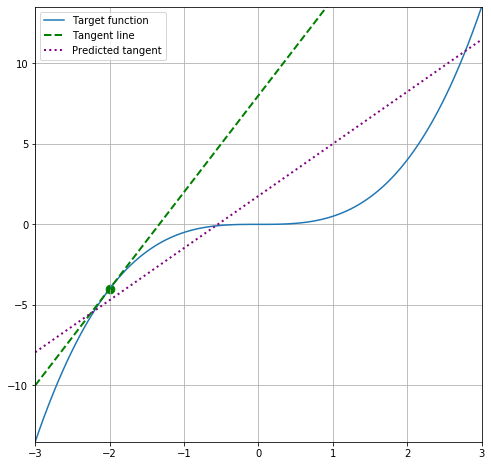Performance is quite off for our target point - apparently, we put a lot of weight on points that are far away (in terms of our measure of proximity) from our target. By slightly adjusting our proximity function, we can reduce those weights on distant datapoints:

$$\tilde{s}(x_i,x_{target};\lambda)=e^{-\frac{||x_i-x_{target}||_2}{\lambda}}$$

Our proximity measure now contains a scaling factor $\lambda>0$ that allows us to adjust the weight we put on datapoints in relation to their distance from our target. This is almost the same as the Squared Exponential Kernel that is often used in Kernel methods and non-parametric statistics. As a side-note, many Kernels define valid measures of proximity that we could use instead of our current one.

By increasing $\lambda$ we put less and less weight on datapoints that are further away from the target. That means that as long as there are sufficiently many datapoints around our target, we can approximate the respective tangent line better and better. If this is not the case and if $\lambda$ is set too large, all weights will be equal to zero and the Linear Regression model will throw an error.

In :
for lambd in [1,10,20,30,40,50]:
similarities_tilde = np.exp(-pairwise_distances(x_sample.reshape(-1,1), target)*lambd)
model_tilde = LinearRegression()
model_tilde.fit(x_sample.reshape(-1,1), y_sample.reshape(-1,1), sample_weight = similarities_tilde.reshape(-1))

print("Predicted value at x={} & lambda = {}: {}".format(target[0,0],lambd,
model_tilde.predict(target)[0,0]))

Predicted value at x=-2 & lambda = 1: -4.706809010226895
Predicted value at x=-2 & lambda = 10: -4.10170754993778
Predicted value at x=-2 & lambda = 20: -4.053528713006144
Predicted value at x=-2 & lambda = 30: -4.0196852557879
Predicted value at x=-2 & lambda = 40: -3.982869957335202
Predicted value at x=-2 & lambda = 50: -3.96915709885981

In :
similarities_tilde = np.exp(-pairwise_distances(x_sample.reshape(-1,1), target)*30)
model_tilde = LinearRegression()
model_tilde.fit(x_sample.reshape(-1,1), y_sample.reshape(-1,1), sample_weight = similarities_tilde.reshape(-1))

Out:
LinearRegression(copy_X=True, fit_intercept=True, n_jobs=None, normalize=False)
In :
plt.figure(figsize = (8,8))
plt.scatter(x_sample, similarities_tilde.reshape(-1))
plt.vlines(-2, ymin = 0, ymax = 1, color = "red", linestyle = "--")

plt.xlim((-3,3))
plt.ylim((0,1))

plt.grid()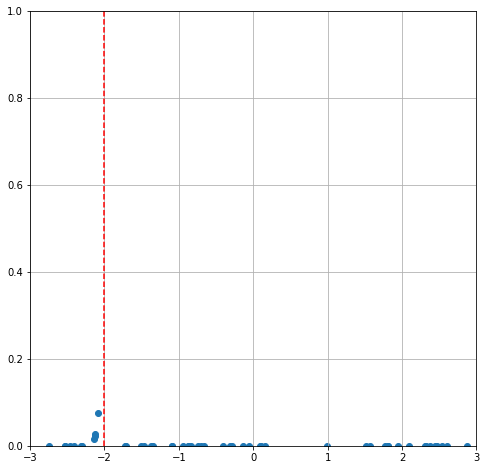In :
np.random.seed(123)

x = np.linspace(-3,3, 100)
y = 0.5*x**3

plt.figure(figsize = (8,8))
plt.plot(x,y, label = "Target function")
plt.plot(x,f2, linestyle = '--', color = "green", lw = 2, label = "Tangent line")
plt.plot(x,model_tilde.predict(x.reshape(-1,1)), linestyle=':', color = "purple", lw=2, label = "Predicted tangent")
plt.scatter(a2, 0.5*a2**3, color = "green", s = 75)

plt.xlim((-3,3))
plt.ylim((np.min(y), np.max(y)))
plt.legend()

plt.grid()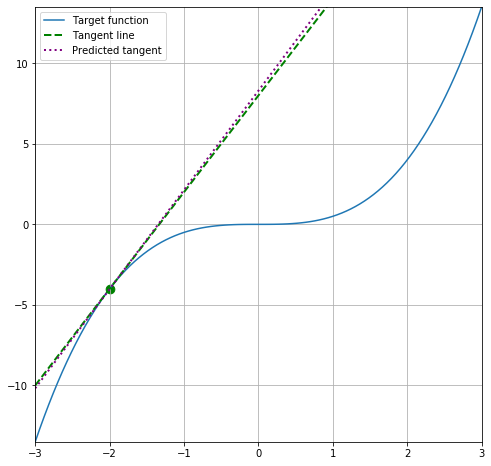With $\lambda=30$ we get a decent result on this toy example. In general for fairly smooth target functions, a higher $\lambda$ should work better and accuracy should increase the more datapoints we have available around the target point. Nevertheless, we typically don't know how smooth the underlying function is and a lower scaling parameter could perform better merely due to a sparse data scatter (have another look at the predictions for increasing $\lambda=40$ and $\lambda=50$). This problem increases further for very high dimensional problems where we might need millions of datapoints before we can reasonably approximate the function in our area of interest.

On the other hand, we now have a locally interpretable, linear model that allows us to interpret the contribution of the feature to the target variable. Additionally, Linear Regression is a fairly sparse model resulting in lower chances of overfitting than with more sophisticated ML solutions.

Probably the most problematic aspect of this approach however is the right choice of proximity measure. Especially for non-continuous features like categorical variables, a continuous measure based on Euclidean Distance might not be the best option. Problems with both categorical and continuous features are even worse and we might need to arbitrarily choose different proximity measures for different dimensions.

In the next part of this mini-series I will therefore show a possible solution to this problem and also some applications on real-world datasets.